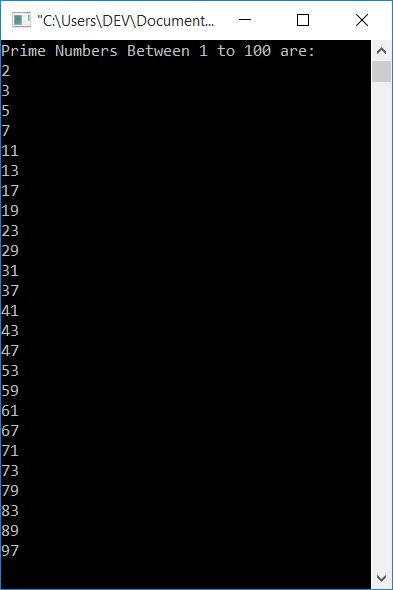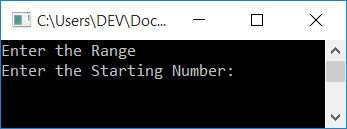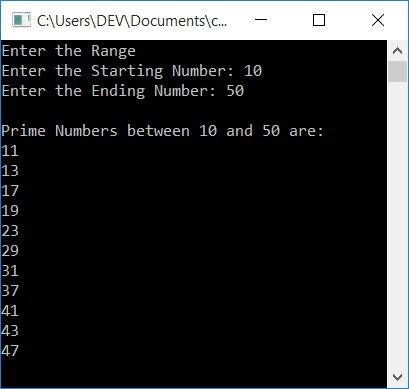# C++ Program to Print Prime Numbers

In this article, you will learn and get code to print prime numbers using a C++ program in the following ways:

Before creating these programs for the printing of prime numbers, let's first understand them.

### What is a prime number?

If a number can't be divisible by any number except 1 and the number itself, then that number is called a prime number. For example, 2, 3, 5, 7, 13, 17, 19, etc.

## Print prime numbers between 1 and 100

This program prints all prime numbers between 1 and 100 using the for loop. The question is, "Write a program in C++ to print prime numbers from 1 to 100." Here is its answer:

```#include<iostream>
using namespace std;
int main()
{
int i, chk=0, j;
cout<<"Prime Numbers Between 1 to 100 are:\n";
for(i=1; i<=100; i++)
{
for(j=2; j<i; j++)
{
if(i%j==0)
{
chk++;
break;
}
}
if(chk==0 && i!=1)
cout<<i<<endl;
chk = 0;
}
cout<<endl;
return 0;
}```

This program was built and runs under the Code::Blocks IDE. Here is its sample output:The dry run of the above program goes like this:

• Initial value, chk=0
• Now 1 gets initialized to i, and the condition i<=100 or 1<=100 evaluates to be true. Therefore, program flow goes inside the loop.
• Inside the loop, there is another for loop, so 2 gets initialized to j, and the condition j<i or 2<1 evaluates to be false, therefore program flow does not go inside this loop's body.
• Now the condition of the if gets evaluated. There are two conditions, and between these two conditions, we've used the && operator. As a result, program flow only execute the if's body statement until both conditions are true.
• But this time, its first condition, which is chk==0 or 0==0, evaluates to be true, but its second condition, which is i!=1 or 1!=1, evaluates to be false, therefore program flow does not go inside its body.
• Now 0 gets initialized to chk, and the program flow goes to the update part of the outer for loop, which increments the value of i. So i=2
• The condition i<=100 or 2<=100 again evaluates to be true, therefore program flow again goes inside the loop.
• 2 gets initialized to j, and the condition j<i or 2<2 evaluates to be false.
• Now the condition of if, that is, chk==0 or 0==0, evaluates to be true, and its second condition, i!=1 or 2!=1 also evaluates to be true. Since both conditions evaluates to be true, program flow goes inside its body, and the value of i gets printed as a prime number, which is 2.
• Now 0 gets initialized to ch, and program flow goes to the update part of the for loop and increments the value of i. So i=3
• The condition i<=100 or 3<=100 again evaluates to be true, therefore program flow again goes inside the loop.
• This process continues until the condition is evaluated as false.
• In this way, prime number gets printed one by one.

## C++ Print Prime Numbers in a Given Range

To print all prime numbers between a particular range (entered by the user) in C++ programming, do a divisibility test (as done in the previous program) using a for loop, from 2 to one less than that number (i.e., n-1). If the number is divided by any number from 2 to one less than that, then the number will not be prime. Otherwise, print it as a prime number, as shown here in the following program.

```#include<iostream>
using namespace std;
int main()
{
int st, en, i, j, chk=0;
cout<<"Enter the Range\n";
cout<<"Enter the Starting Number: ";
cin>>st;
cout<<"Enter the Ending Number: ";
cin>>en;
cout<<"\nPrime Numbers between "<<st<<" and "<<en<<" are:\n";
for(i=st; i<=en; i++)
{
for(j=2; j<i; j++)
{
if(i%j==0)
{
chk++;
break;
}
}
if(chk==0 && i!=1)
cout<<i<<endl;
chk = 0;
}
cout<<endl;
return 0;
}```

Here is the initial output of this program's sample run:Now enter the two numbers, say 10 and 50, as the starting and ending numbers to print all prime numbers between 10 and 50, as shown in the screenshot below:#### The same program in different languages

C++ Quiz

« Previous Program Next Program »